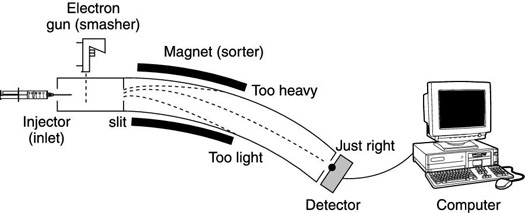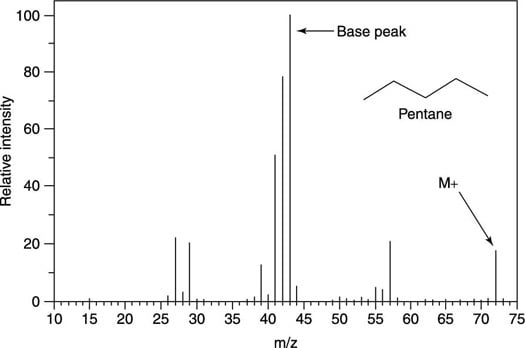##### Organic Chemistry I For DummiesA mass spectrometer can help you locate unknown ions in the mass spectrum so that you can identify them. When charged particles move through the magnetic field of a mass spectrometer, they're deflected (pulled off course) by the magnetic field; they then hit the detector, as the first figure shows.The inside of a mass spectrometer.

The number of ions that bend the right amount at a particular magnetic field strength to hit the detector is measured. On a mass spectrum, the molecular weight of the fragment (m/z) is shown on the x-axis versus the relative number of fragments that hit the detector on the y-axis (the intensity). The most intense peak on the mass spectrum is arbitrarily assigned an intensity value of 100. The next figure shows the mass spectrum for pentane.The mass spectrum for pentane (C5H12).

It's actually not the weight of the fragment that's measured on the x-axis of the mass spectrum, but the mass (m) to charge (z) ratio (called the m/z value). A fragment that has a +2 charge will require a smaller magnetic field to deflect it toward the detector than a fragment that has a +1 charge, and will therefore show up on the mass spectrum at half the m/z value. However, since most fragments simply have charges of +1 (and since the vast majority of undergraduate classes will not discuss fragments with more than one charge), the m/z axis is often simply said to represent the molecular weight of the fragment. That is, given that the mass-to-charge ratio is m/z, and the charge (z) equals +1, in most cases, m/z = m/1 = m.

Referring again to the mass spectrum for the molecule pentane (C5H12), this spectrum contains several peaks that are worth pointing out. The molecular ion peak, or M+ peak, is the peak at m/z 72, which represents the molecular weight of the molecule (72 amu). The tallest peak in the spectrum, which is always given a value on the y-axis of 100, is called the base peak. The base peak represents the most abundant fragment that hits the detector. In some spectra, the base peak is also the M+ peak, although in this case it's not.

The mass spectrum for pentane is fairly simple, but it still contains a lot of peaks, each of which represents a different fragment that was formed by electron ionization. Usually, you can't expect to be able to identify all the peaks in a mass spectrum (or even most of them).